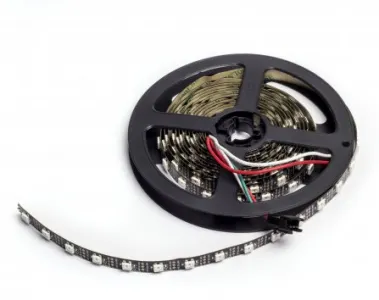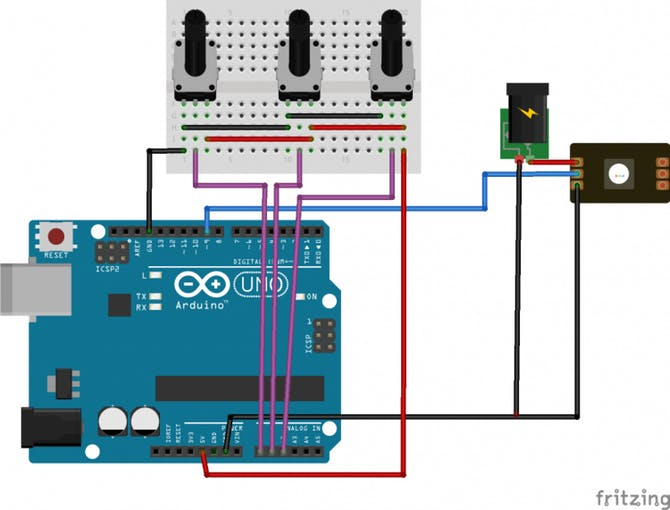Project showcaseThis lesson aims to show how to control an addressable WS2812 LEDs strip in order to obtain various colors using 3 potentiometers.

• 6,670 views
• 1 comment
• 8 respects

## Components and suppliesArduino UNO & Genuino UNO
×1
 WS2811 Addressable LED strip (1m, 60 LEDs)
×1
 10 10K trimmer
×1

### 2. How does it work?

The sketch is very simple: there are 3 potentiometers attached to three different analog inputs (A0, A1 and A2). Each one control the amount of color (Red Green and Blue respectively) in 1 meter of addressable WS2812 LEDs.

For sake of simplicity in the following layout only one LED is reported.

Attention: Please make sure to supply the strip using an external 5V power supply as reported in the layout!

### 3. Code

Here the code! Upload it and start to play with colors!

``````/*
Parts required:
1m strip of addressable LEDs http://store.arduino.cc/products/C000083
Created 28 November 2014
This example code is part of the public domain
*/
const int NUMPIXELS = 60; //number of LEDs in 1m
const int LEDsPin = 9;    // LEDs connected to digital pin 9
const int redPotPin = A0;      // pin to control red
const int greenPotPin = A1;   // pin to control green
const int bluePotPin = A2;   // pin to control blue
int redValue = 0; // value to write to the red LED
int greenValue = 0; // value to write to the green LED
int blueValue = 0; // value to write to the blue LED
int redPotValue = 0; // variable to hold the value from the red pot
int greenPotValue = 0; // variable to hold the value from the green pot
int bluePotValue = 0; // variable to hold the value from the blue pot
// When we setup the NeoPixel library, we tell it how many pixels, and which pin to use to send signals.
void setup() {
// initialize serial communications at 9600 bps:
Serial.begin(9600);
// set the digital pin as output
pinMode(LEDsPin, OUTPUT);
}
void loop() {
// read the value from the red pot control:
// give the ADC a moment to settle
delay(5);
// read the value from the green pot control:
// give the ADC a moment to settle
delay(5);
// read the value from the blue pot control:
// print out the values to the serial monitor
Serial.print("raw sensor Values \t red: ");
Serial.print(redPotValue);
Serial.print("\t green: ");
Serial.print(greenPotValue);
Serial.print("\t Blue: ");
Serial.println(bluePotValue);
/*
In order to use the values from the pots for the LEDs,
you need to do some math. The ADC provides a 10-bit number,
but analogWrite() uses 8 bits. You'll want to divide your
sensor readings by 4 to keep them in range of the output.
*/
redValue = map(redPotValue, 0, 1023, 0, 255);
greenValue = map(greenPotValue, 0, 1023, 0, 255);
blueValue = map(bluePotValue, 0, 1023, 0, 255);;
//  print out the mapped values
Serial.print("Mapped sensor Values \t red: ");
Serial.print(redValue);
Serial.print("\t green: ");
Serial.print(greenValue);
Serial.print("\t Blue: ");
Serial.println(blueValue);
// For a set of NeoPixels the first NeoPixel is 0, second is 1, all the way up to the count of pixels minus one.
for (int i = 0; i < NUMPIXELS; i++) {
// pixels.Color takes RGB values, from 0,0,0 up to 255,255,255
pixels.setPixelColor(i, pixels.Color(redValue, greenValue, blueValue));
pixels.show(); // This sends the updated pixel color to the hardware.
delay(50); // Delay for a period of time (in milliseconds).
}
}
``````

## Code

##### Code snippet #1Arduino
```/*

Parts required:
1m strip of addressable LEDs http://store.arduino.cc/products/C000083

This example code is part of the public domain
*/

const int NUMPIXELS = 60; //number of LEDs in 1m
const int LEDsPin = 9;    // LEDs connected to digital pin 9

const int redPotPin = A0;      // pin to control red
const int greenPotPin = A1;   // pin to control green
const int bluePotPin = A2;   // pin to control blue

int redValue = 0; // value to write to the red LED
int greenValue = 0; // value to write to the green LED
int blueValue = 0; // value to write to the blue LED

int redPotValue = 0; // variable to hold the value from the red pot
int greenPotValue = 0; // variable to hold the value from the green pot
int bluePotValue = 0; // variable to hold the value from the blue pot

// When we setup the NeoPixel library, we tell it how many pixels, and which pin to use to send signals.

void setup() {
// initialize serial communications at 9600 bps:
Serial.begin(9600);

// set the digital pin as output
pinMode(LEDsPin, OUTPUT);
}

void loop() {

// read the value from the red pot control:
// give the ADC a moment to settle
delay(5);
// read the value from the green pot control:
// give the ADC a moment to settle
delay(5);
// read the value from the blue pot control:

// print out the values to the serial monitor
Serial.print("raw sensor Values \t red: ");
Serial.print(redPotValue);
Serial.print("\t green: ");
Serial.print(greenPotValue);
Serial.print("\t Blue: ");
Serial.println(bluePotValue);

/*
In order to use the values from the pots for the LEDs,
you need to do some math. The ADC provides a 10-bit number,
but analogWrite() uses 8 bits. You'll want to divide your
sensor readings by 4 to keep them in range of the output.
*/
redValue = map(redPotValue, 0, 1023, 0, 255);
greenValue = map(greenPotValue, 0, 1023, 0, 255);
blueValue = map(bluePotValue, 0, 1023, 0, 255);;

//  print out the mapped values
Serial.print("Mapped sensor Values \t red: ");
Serial.print(redValue);
Serial.print("\t green: ");
Serial.print(greenValue);
Serial.print("\t Blue: ");
Serial.println(blueValue);

// For a set of NeoPixels the first NeoPixel is 0, second is 1, all the way up to the count of pixels minus one.
for (int i = 0; i < NUMPIXELS; i++) {
// pixels.Color takes RGB values, from 0,0,0 up to 255,255,255
pixels.setPixelColor(i, pixels.Color(redValue, greenValue, blueValue));
pixels.show(); // This sends the updated pixel color to the hardware.
delay(50); // Delay for a period of time (in milliseconds).
}
}
```

## SchematicsJuly 4, 2016

#### Members who respect this project

See similar projects
you might like

#### Arduino Color Mixer

Project tutorial by Ahmed Hamdy

• 20,034 views
• 66 respects

#### Color Sensor Prototype: Detect Color Name from Objects

Project tutorial by moretticb

• 8,084 views
• 33 respects

• 10,446 views
• 11 respects

#### Using SMS messages to control LED color

Project tutorial by SteiniBrodda

• 9,207 views
• 6 respects

#### Arduino RGB Color Mixer

Project tutorial by FIELDING

• 8,414 views